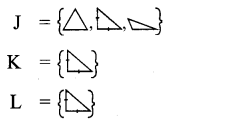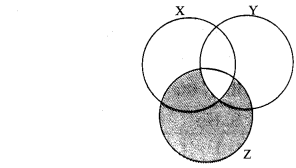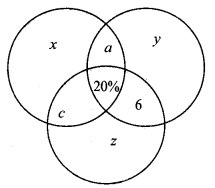PRINTABLE FOR KIDS

XII (12) HSC

XI (11) FYJC
X (10) SSC

## Tamilnadu Samacheer Kalvi 9th Maths Solutions Chapter 1 Set Language Ex 1.7

Multiple Choice Questions
Question 1.
Which of the following is correct?
(1) {7} ∈ {1, 2, 3, 4, 5, 6, 7, 8, 9, 10}
(2) 7 ∈ {1, 2, 3, 4, 5, 6, 7, 8, 9, 10}
(3) 7 ∉ {1, 2, 3, 4, 5, 6, 7, 8, 9, 10}
(4) {7} ⊈ {1, 2, 3, 4, 5, 6, 7, 8, 9, 10}
Solution:
(2) 7 ∈ {1, 2, 3, 4, 5, 6, 7, 8, 9, 10}

Question 2.
The set P = {x | x ∈ Z, -1 < X < 1} is a
(1) Singleton set
(2) Power set
(3) Null set
(4) Subset
Solution:
(1) Singleton set
Hint: P = {0}

Question 3.
If U = {x | x ∈ N, x < 10} and A = {x | x ∈ N, 2 ≤ x < 6} then (A’)’ is
(1) {1, 6, 7, 8, 9}
(2) {1, 2, 3, 4}
(3) {2, 3, 4, 5}
(4) { }
Solution:
(3) {2, 3, 4, 5}
Hint: (A’) = A= {2, 3, 4, 5}

Question 4.
If B ⊆ A then n(A ∩ B) is
(1) n(A – B)
(2) n(B)
(3) n(B – A)
(4) n(A)
Solution:
(2) n(B)
Hint: B ⊆ A ⇒ A ∩ B = B

Question 5.
If A= {x, y, z} then the number of non-empty subsets of A is
(1) 8
(2) 5
(3) 6
(4) 7
Solution:
(4) 7
Hint: Number of non-empty subsets = 2 – 1 = 8 – 1 = 7

Question 6.
Which of the following is correct ?
(1) Ø ⊆ {a,b}
(2) Ø ∈ {a, b}
(3) {a} ∈ {a, b}
(4) a ⊆ {a, b}
Solution:
(1) Ø ⊆ {a,b}
Hint: Empty set is an improper subset

Question 7.
If A ∪ B = A ∩ B, then
(1) A ≠ B
(2) A = B
(3) A ⊂ B
(4) B ⊂ A
Solution:
(2) A = B

Question 8.
If B – A is B, then A ∩ B is
(1) A
(2) B
(3) U
(4) Ø
Solution:
(4) Ø
Hint: B – A = B ⇒ A and B are disjoint sets.

Question 9.
From the adjacent diagram n[P(A ∆ B) is
(1) 8
(2) 16
(3) 32
(4) 64

Solution:
(3) 32
Hint: A ∆ B = { 60, 85, 75, 90, 70}
⇒ n(A ∆ B) = 5
⇒ n(P(A ∆ B)) = 25 = 32

Question 10.
If n(A) = 10 and n(B) = 15 then the minimum and maximum number of elements in A ∩ B is
(1) (10, 15)
(2) (15, 10)
(3) (10, 0)
(4) (0, 10)
Solution:
(4) (0, 10)

Question 11.
Let A = {Ø} and B = P(A) then A ∩ B is
(1) {Ø, {Ø} }
(2) {Ø}
(3) Ø
(4) {0}
Solution:
(2) {Ø}
Hint: P(A) = {Ø {Ø}}

Question 12.
In a class of 50 boys, 35 boys play carom and 20 boys play chess then the number of boys play both games is
(1) 5
(2) 30
(3) 15
(4) 10
Solution:
(1) 5
Hint: n(A ∪ B) = n(A) + n(B) – n(A n B) ⇒ 50 = 35 + 20 – n(A ∩ B) ⇒ n(A ∩ B) = 5

Question 13.
If U = {x : x ∈ N and x < 10}, A = {1, 2, 3, 5, 8} and B = {2, 5, 6, 7, 9}, then n[(A ∪ B)’] is
(1) 1
(2) 2
(3) 4
(4) 8
Solution:
(1) 1
Hint: U = {1, 2, 3, 4, 5, 6, 7, 8, 9}
A = {1, 2, 3, 5, 8}
B = {2, 5, 6, 7, 9}
A ∪ B = {1, 2, 3, 5, 6, 7, 8, 9}
(A ∪ B)’ = {4},
n(A ∪ B)’ = 1

Question 14.
For any three sets P, Q and R, P – (Q ∩ R) is
(1), P – (Q ∪ R)
(2) (P ∩ Q) – R
(3) (P – Q) ∪ (P – R)
(4) (P – Q) ∩ (P – R)
Solution:
(3) (P – Q) ∪ (P – R)
Hint: P – (Q ∩ R) = (P – Q) ∪ (P – R)

Question 15.
Which of the following is true?
(1) A – B = A ∩ B
(2) A – B = B – A
(3) (A ∪ B)’ = A’ ∪ B’
(4) (A ∩ B)’ = A’ ∪ B’
Solution:
(4) (A ∩ B)’ = A’ ∪ B’

Hint: (1) (A – B) = A ∩ B ✘
(2) A – B = B – A ✘
(3) (A ∪ B) = A’ ∪ B’ ✘
(4) (A ∩ B)’ = A’ ∪ B’ ✓

Question 16.
If n(A ∪ B ∪ C) = 100, n(A) = 4x, n(B) = 6x, n(C) = 5x, n(A ∩ B) = 20, n(B ∩ C) = 15, n(A ∩ C) = 25 and n(A ∩ B ∩ C)= 10 , then the value of x is
(A) 10
(B) 15
(C) 25
(D) 30
Solution:
(A) 10
Hint:
n(A ∪ B ∪ C) = n(A) + n(B) + n(C) – n(A ∩ B) – n(B ∩ C) – n(C ∩ A) + n(A ∩ B ∩ C)
100 = 4x + 6x + 5x – 20 – 15 – 25 + 10
100 = 15x -60 + 10
100 = 15x – 50
∴ 15x = 100 + 50 = 150
x = 10

Question 17.
For any three sets A, B and C, (A – B) ∩ (B – C) is equal to
(1) A only
(2) B only
(3) C only
(4) ϕ
Solution:
(4) ϕ
Hint: (A – B) ∩ (B – C) is equal to Φ

Question 18.
If J = Set of three sided shapes, K = Set of shapes with two equal sides and L = Set of shapes with right angle, then J ∩ K ∩ L is
(1) Set of isoceles triangles
(2) Set of equilateral triangles
(3) Set of isoceles right triangles
(4) Set of right angled triangles
Solution:
(3) Set of isoceles right triangles
Hint:Question 19.
The shaded region in the Venn diagram is
(1) Z – (X ∪ Y)
(2) (X ∪ Y) ∩ Z
(3) Z – (X ∩ Y)
(4) Z ∪ (X ∩ Y)
(3) Z – (X ∩ Y)
Hint:Z – (X ∩ Y)

Question 20.
In a city, 40% people like only one fruit, 35% people like only two fruits, 20% people like all the three fruits. How many percentage of people do not like any one of the above three fruits?
(1) 5
(2) 8
(3) 10
(4) 15
(1) 5
Hint:40 + 35 + 20 + x = 100%
95% + x = 100%
x = 5%

## PDF FILE TO YOUR EMAIL IMMEDIATELY PURCHASE NOTES & PAPER SOLUTION. @ Rs. 50/- each (GST extra)

SUBJECTS

HINDI ENTIRE PAPER SOLUTION

MARATHI PAPER SOLUTION
SSC MATHS I PAPER SOLUTION
SSC MATHS II PAPER SOLUTION
SSC SCIENCE I PAPER SOLUTION
SSC SCIENCE II PAPER SOLUTION
SSC ENGLISH PAPER SOLUTION
SSC & HSC ENGLISH WRITING SKILL
HSC ACCOUNTS NOTES
HSC OCM NOTES
HSC ECONOMICS NOTES
HSC SECRETARIAL PRACTICE NOTES

2019 Board Paper Solution

HSC ENGLISH SET A 2019 21st February, 2019

HSC ENGLISH SET B 2019 21st February, 2019

HSC ENGLISH SET C 2019 21st February, 2019

HSC ENGLISH SET D 2019 21st February, 2019

SECRETARIAL PRACTICE (S.P) 2019 25th February, 2019

HSC XII PHYSICS 2019 25th February, 2019

CHEMISTRY XII HSC SOLUTION 27th, February, 2019

OCM PAPER SOLUTION 2019 27th, February, 2019

HSC MATHS PAPER SOLUTION COMMERCE, 2nd March, 2019

HSC MATHS PAPER SOLUTION SCIENCE 2nd, March, 2019

SSC ENGLISH STD 10 5TH MARCH, 2019.

HSC XII ACCOUNTS 2019 6th March, 2019

HSC XII BIOLOGY 2019 6TH March, 2019

HSC XII ECONOMICS 9Th March 2019

SSC Maths I March 2019 Solution 10th Standard11th, March, 2019

SSC MATHS II MARCH 2019 SOLUTION 10TH STD.13th March, 2019

SSC SCIENCE I MARCH 2019 SOLUTION 10TH STD. 15th March, 2019.

SSC SCIENCE II MARCH 2019 SOLUTION 10TH STD. 18th March, 2019.

SSC SOCIAL SCIENCE I MARCH 2019 SOLUTION20th March, 2019

SSC SOCIAL SCIENCE II MARCH 2019 SOLUTION, 22nd March, 2019

XII CBSE - BOARD - MARCH - 2019 ENGLISH - QP + SOLUTIONS, 2nd March, 2019

HSC Maharashtra Board Papers 2020

(Std 12th English Medium)

HSC ECONOMICS MARCH 2020

HSC OCM MARCH 2020

HSC ACCOUNTS MARCH 2020

HSC S.P. MARCH 2020

HSC ENGLISH MARCH 2020

HSC HINDI MARCH 2020

HSC MARATHI MARCH 2020

HSC MATHS MARCH 2020

SSC Maharashtra Board Papers 2020

(Std 10th English Medium)

English MARCH 2020

HindI MARCH 2020

Hindi (Composite) MARCH 2020

Marathi MARCH 2020

Mathematics (Paper 1) MARCH 2020

Mathematics (Paper 2) MARCH 2020

Sanskrit MARCH 2020

Important-formula

THANKS